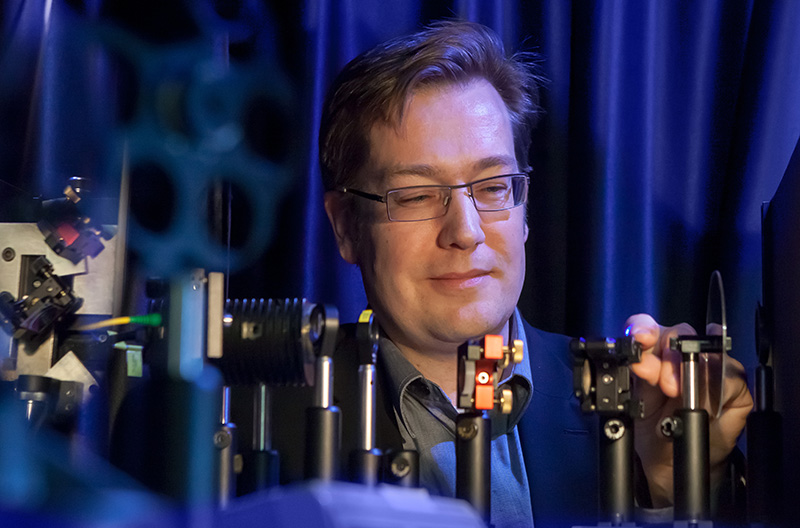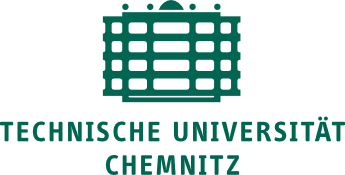Some links collected over the last months.

I will be at the ISCPAC 2016 meeting next week. In case you are also there, meet up:-)

[2016-06-07 Some Updates in the afternoon;-)]

The diode ideality factor in organic solar cells: basics

Where does one start after so long an absence — meaning only the blog abstinence; I have been working and publishing since last time;-)One of the things which have been on my mind is the ideality factor, a figure of merit for the charge carrier recombination mechanism in a semiconductor diode. In short, a diode ideality factor of 1 is interpreted as direct recombination of electrons and holes across the bandgap. An ideality factor of 2 is interpreted as recombination through defects states, i.e. recombination centres. More on that in a later post, let’s start with the basics.

A couple of years ago, I wrote about some general properties of current-voltage characteristics of organic solar cells, but did not describe the ideality factor.1 I think the ideality factor was mentioned only once, and then without details.

The Shockley diode equation describes the current–voltage characteristics of a diode,$j=j_0 \left(\exp\left(\frac{eV}{n_{id}kT}\right)-1\right) - j_{gen}$.

Here,$j$ current,$V$ the voltage,$e$ elementary charge,$kT$ thermal voltage,$j_0$ the dark saturation current, and$j_{gen}$ the photogenerated current. If the ideality factor$n_{id}$ was equal to one, one could call this the ideal Shockley equation. It derivation can be found in semiconductor text books, but it can also be derived based on thermodynamic arguments (see Peter Würfel’s excellent book on the physics of solar cells).

The current$j$ flowing out of the diode is defined to be negative. Essentially, the charge carriers which can flow out are the generated ones (e.g.$j_{gen}$), but reduced by the recombination current. That means,$j=\underbrace{j_0 \left(\exp\left(\frac{eV}{n_{id}kT}\right)-1\right)}_{j_{rec}} - j_{gen}$.

However, the term$j_{rec}$ contains also a negative contribution,$j_0$ times the$-1$ from the bracket. This is the thermal generation current$j_{gen,th} \equiv j_0$, i.e. charge carriers excited across the bandgap just by thermal energy — and therefore very little. Still, the term is very important, as it is the prefactor of the whole$j(V)$ curve. Without light, i.e. with photocurrent$j_{gen}=0$, we can clarify$j=\underbrace{j_0 \exp\left(\frac{eV}{n_{id}kT}\right)}_{j_{rec,dark}} - \underbrace{j_0}_{j_{gen,th}}$.

so that at negative voltages,$j=-j_0$.(Please note that under realistic conditions,$j_0$ is not only pretty small and difficult to measure in principle, it is also hidden behind shunt currents in the device. ) At zero volt,$j=j_0-j_0=0$. Thus, generation = recombination — or more specifically, thermal generation current = recombination current — which essentially implies that 0V correspond to the open circuit voltage in the dark.

How can one determine the ideality factor and the dark saturation current (at least in principle, see below for a better way on real devices)? It is common to neglect the thermal generation current (the term -1, multiplied by$j_0$), which is a good approximation for voltages some$kT/e$ larger than 0. Then, calculate the logarithm of the dark current ($j_{gen}=0$),$\ln(j) = \ln(j_0) +\frac{e}{n_{id}kT}V$,

so that the ideality factor can be determined from the inverse slope of the ln(current) at forward bias, and the dark saturation current from the current-axis offset. Let me already tell you that I do not recommend this approach, for reasons written below, and as explained in more detail in a recent paper of Kris Tvingstedt and myself [Tvingstedt/Deibel 2016].

Under illumination and at open circuit conditions,$j(V_{oc})=0$, we can rewrite the Shockley equation as$j_{gen}=j_0 \left(\exp\left(\frac{eV_{oc}}{n_{id}kT}\right)-1\right)$,

which has the same shape as the Shockley equation in the dark. This means that if you measure ($j_{gen}, V_{oc}$) pairs for a (wide) range of different illumination intensities (thus varying$j_{gen}$), the points should overlap with the dark$j(V)$ curve! We’ll come back to this important point further below. Note that for solar cells with good fill factor,$j_{gen}$ can be approximated by the short circuit current$j_{sc}$. Continue reading “The diode ideality factor in organic solar cells: basics”

Interaction of light with solids in experiment and simulation

Hi there, sorry for not getting back to you but starting a new group and having new responsibilities (e.g. involvement in new degree programmes for Material Science) can take (part of) the blame.Just as brief progress indicator, here a link to an interview of the Chemnitz University of Technology press office with me. (Photo: Uwe Meinhold)

The official short name of my group is OPKM, for Optics and Photonics of Condensed Matter. For the (very) long official name I refer you to the web page of the Institute of Physics at the TUC;-) The size of my group is growing slowly but steadily, and the lab building shows progress as well: setups for time correlated single photon counting to measure photoluminescence transients – e.g. to determine charge carrier recombination in perovskite solar cells – and for confocal measurements of luminescence are already available from my predecessor’s group: we just adapt them to our needs. Other setups, time resolved and steady state, are being built and come along nicely. Solar cell preparation is still improvised, using the glovebox system of a colleague and the evaporation chamber of another, until we get our own integrated glovebox/evaporator system. One of my main interests is still Organic Photovoltaics, and with my (PhD) background in inorganic photovoltaics I also look at the hybrid perovskite solar cell hype (as a hype is not necessarily a bad thing;-). What also remains is my joy to combine experiments and simulations (macroscopic device simulations, kinetic Monte Carlo simulations) to understand these systems.

If you are interested in joining us: I have two PhD positions available at present. Please check out the job offer (german; computer-translated here) and contact me.

Cheers!

Restarting in Chemnitz

Just a brief note,I moved from Würzburg to the Institute of Physics at Chemnitz University of Technology this March, starting a new group. At present I have one PhD position open on Organic Photovoltaics – funded by the University, therefore including some teaching duty in German. Have a look here (in German) or drop me a line if you are interested. Cheers,

Carsten

Some links I found interesting since the last time… partly older stuff, though.

Nongeminate recombination in organic solar cells – slower than expected

In a “recent” post (just 3 posts but 10 months ago;-) I wrote once again on the derivation of the Langevin recombination rate for nongeminate recombination.The question is, is Langevin recombination really what governs the charge carrier loss rate in organic solar cells?

Recombination of electrons with holes is usually a 2nd order decay. As electrons$n$ and holes$p$ are photogenerated pair wise, the respective excess charge carrier concentrations are symmetric,$n=p$. Then a recombination rate$R$ is$R = k n p = k n^2$,

where the recombination prefactor$k$ could be a Langevin prefactor – more on that later. In a transient experiment with a photogenerating, short laser pulse at$t=0$, the continuity equation for charge carriers (here, e.g. electrons) under open circuti conditions (no external current flow, for instance if the experiment is done on a thin film without electrodes)$\frac{dn}{dt} = G-R$

becomes$\frac{dn}{dt} = -R$

for$t>0$ (as the generation was only at$t=0$).

If all electrons and hole are available for recombination (i.e., can reach all other charge carriers and can be reached by them), then the recombination rate and the continuity equation for$t>0$ yield$\frac{dn}{dt} = k n^2$ Continue reading “Nongeminate recombination in organic solar cells – slower than expected”

New life – again

On 11th of February (my birthday, incidentally), our 2nd child was born: Nicolas Jacob. We are so happy, as is our 2 year old daughter Chiara.

Even with only one kid, it was already rather quiet on this blog in the last months (well, 2 years…). Still, I do have some hope to be able to dedicate one or the other quiet minute to write some urgently needed updates to previous posts. All the best, Carsten

Belectric aquires German Konarka Daughter

Related to this post on Konarka’s bankruptcy: According to a range of news sites,including pv-tech.org, the german company Belectric has acquired Konarka Technologies. Find the press release here (pdf).

The system integrator Belectric is situated in Lower Franconia, less than 50km from Würzburg and less than 10km from where I live. Let’s keep our fingers crossed!

Nongeminate Recombination: Langevin (again) and beyond (later;-)

Nongeminate recombination is the major loss mechanism for state-of-the-art organic solar cells. In an early blog post, I showed how the Langevin recombination was derived.Although there is more to nongeminate recombination than just this mechanism, it is still instructive and also relevant to trap-assisted recombination mechanisms, due to its mobility-containing prefactor.

[Nenashev 2010] pointed out that in the derivation of the Langevin recombination,

since the electric field scales as r−2 and the surface area of the sphere scales as 2, the value of r chosen is unimportant, leading to a simple solution with constant electron den- sity, thus justifying the neglect of diffusion.

Konarka bankrupt

Via Juan Bisquert’s post: solar cell company Konarka filed for bankrupty yesterday according to Businessweek. It is always hard to be the first… Konarka received its first venture capital in mid 2001.

Howard Berke, CEO of Konarka:

This is a tragedy for Konarka’s shareholders and employees and for the development of alternative energy in the U.S.

Let’s hope that our friends from Konarka find other suitable positions, and that other companies such as Heliatek take up the lead!